## 14.6.2 Plan and Transform

For the decoupled approach in this section, assume that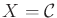, which means there are only velocity constraints, as covered in Section 13.1. The system may be specified as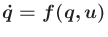or implicitly as a set of constraints of the form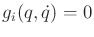. The ideas in this section can easily be extended to phase spaces. The method given here was developed primarily by Laumond (see ) and was also applied to the simple car of Section 13.1.2 in ; other applications of the method are covered in .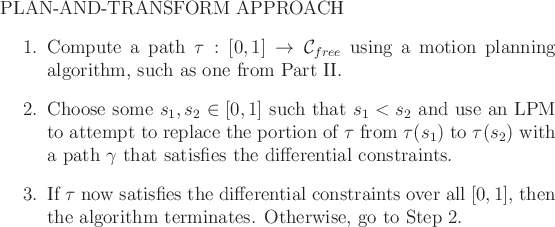An outline of the plan-and-transform approach is shown in Figure 14.21. In the first step, a collision-free path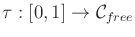is computed by ignoring differential constraints. The path is then iteratively modified until it satisfies the constraints. In each iteration, a subinterval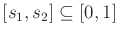is selected by specifying some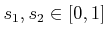so that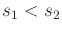. These points may be chosen using random sequences or may be chosen deterministically. The approach may use binary subdivision to refine intervals and gradually improve the resolution on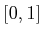over the iterations.

For each chosen interval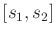, an LPM is used to compute a path segment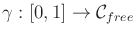that satisfies the conditions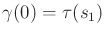and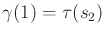. It might be the case that the LPM fails because it cannot connect the two configurations or a collision may occur. In this case, another subinterval is chosen, and the process repeats. Each time the LPM succeeds,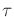is updated to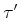as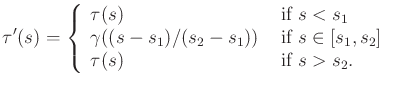(14.30)

The argument to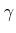reparameterizes it to run from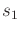to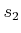, instead of 0 to.

Example 14..5 (Plan-and-Transform for the Dubins Car)   For a concrete example, suppose that the task is to plan a path for the Dubins car. Figure 14.22 shows a paththat might be computed by a motion planning algorithm that ignores differential constraints. Two sharp corners cannot be traversed by the car. Suppose thatandare chosen at random, and appear at the locations shown in Figure 14.22. The portion ofbetween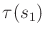and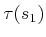needs to be replaced by a path that can be executed by the Dubins car. Note that matching the orientations atandis important because they are part of the configuration.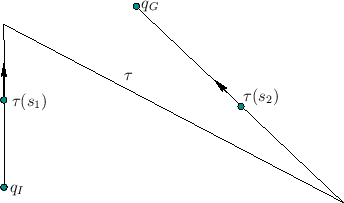A replacement pathis shown in Figure 14.23. This is obtained by implementing the following LPM. For the Dubins car, a path between any configurations can be found by drawing circles at the starting and stopping configurations as shown in the figure. Each circle corresponds to the sharpest possible left turn or right turn. It is straightforward to find a line that is tangent to one circle from each configuration and also matches the direction of flow for the car (the circles are like one-way streets). Using, the pathis updated to obtain, which is shown in Figure 14.24, and satisfies the differential constraints for the Dubins car. This problem was very simple, and in practice dozens of iterations may be necessary to replace path segments. Also, if randomization is used, then intervals of the form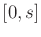and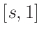must not be neglected.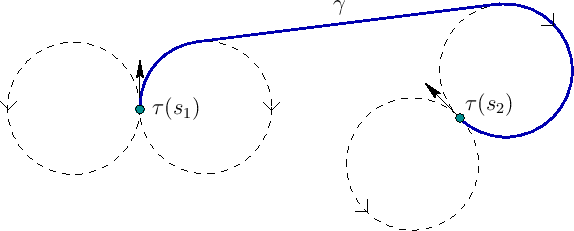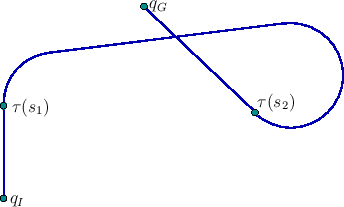Example 14.5 seemed easy because of the existence of a simple local planner. Also, there were no obstacles. Imagine thatinstead traveled through a narrow, zig-zagging corridor. In this case, a solution might not even exist because of sharp corners that cannot be turned by the Dubins car. If there had been an single obstacle that happened to intersect the loop in Figure 14.24, then the replacement would have failed. In general, there is no guarantee that the replacement segment is collision-free. It is important for the LPM to construct path segments that are as close as possible to the original path. For the Dubins car, this is not possible in many cases. For example, moving the Dubins car a small distance backward requires moving along the circles shown in Figure 14.23. Even as the distance between two configurations is reduced, the distance that the car needs to travel does not approach zero. This is true even if the shortest possible paths are used for the Dubins car.

What property should an LPM have to ensure resolution completeness of the plan-and-transform approach? A sufficient condition is given in . Let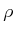denote a metric on. An LPM is said to satisfy the topological property if and only if the following statement holds: For any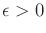, there exists some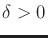such that for any pair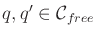having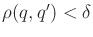implies that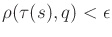for all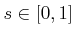. If an LPM satisfies the topological property, then any collision-free path throughcan be transformed into one that satisfies the differential constraints. Suppose that a pathhas some clearance of at least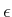in. By dividing the domain ofinto intervals so that the change in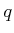is no more than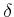over each interval, then the LPM will produce collision-free path segments for replacement.

It turns out that for the Reeds-Shepp car (which has reverse) such an LPM can be designed because it is small-time locally controllable, a property that will be covered in Sections 15.1.3 and 15.4. In general, many techniques from Chapter 15 may be useful for analyzing and designing effective LPMs.

An interesting adaptation of the plan-and-transform approach has been developed for problems that involveimplicit constraints of the form. An outline of the multi-level approach, which was introduced in , is shown in Figure 14.25 (a similar approach was also introduced in ). The idea is to sort theconstraints into a sequence and introduce them one at a time. Initially, a path is planned that ignores the constraints. This path is first transformed to satisfy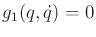and avoid collisions by using the plan-and-transform method of Figure 14.21. If successful, then the resulting path is transformed into one that is collision-free and satisfies bothand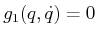. This process repeats by adding one constraint each time, until either the method fails or allconstraints have been taken into account.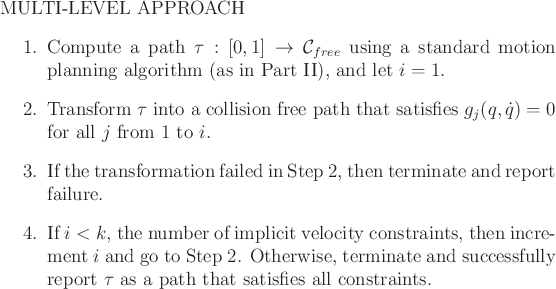Steven M LaValle 2020-08-14